Short Answer Questions: Chemical Reactions & Equations

# Short Answer Questions: Chemical Reactions & Equations - Science Class 10

Q1: What is the role of a catalyst in a chemical reaction?
Ans: A catalyst is a substance that speeds up a chemical reaction but is not consumed in the reaction. It provides an alternative reaction pathway with a lower activation energy, thus increasing the rate of reaction. Catalysts can be specific for certain reactions and do not alter the final products of the reaction. For instance, enzymes are biological catalysts that speed up reactions in living organisms.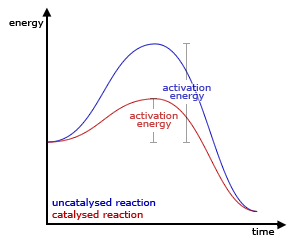Higher activation energy when the reaction is catalyzed

Q2: What is a precipitation reaction? Give an example.
Ans: A precipitation reaction is a type of chemical reaction where two soluble salts in aqueous solution combine to form an insoluble salt (precipitate). This precipitate can be separated from the solution by filtration. For example, when solutions of silver nitrate and sodium chloride are mixed, the insoluble salt silver chloride forms and precipitates out of the solution: AgNO3(aq) + NaCl(aq) → AgCl(s) + NaNO3(aq).

Q3: What is a redox reaction?
Ans: A redox (reduction-oxidation) reaction is a type of chemical reaction that involves a transfer of electrons between two species. An oxidation reaction is one in which a substance loses electrons, while a reduction reaction is one in which a substance gains electrons. For example, in the reaction between hydrogen and oxygen to form water, hydrogen is oxidized (loses electrons) and oxygen is reduced (gains electrons): 2H2 + O2 → 2H2O.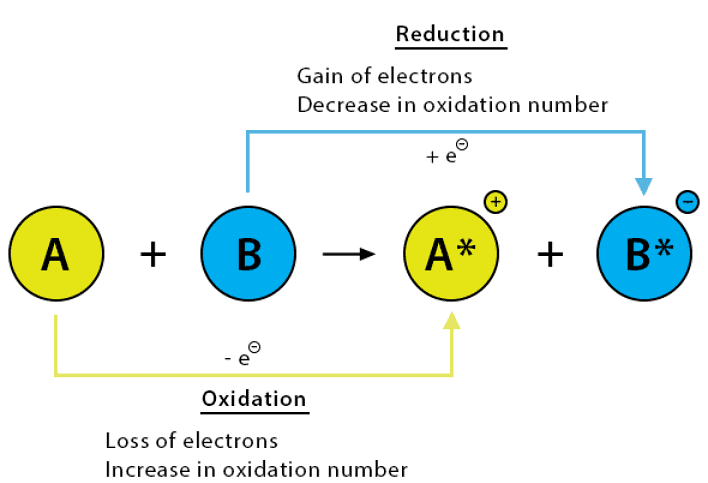How Redox reaction happens

Q4: What is a chemical reaction?
Ans: A chemical reaction refers to the process that leads to the transformation of one set of chemical substances into another. It involves the making or breaking of bonds between atoms, ions, or molecules. During a chemical reaction, the reactants are transformed into products.

For instance, when iron rusts, the iron (reactant) reacts with oxygen to form iron oxide (product).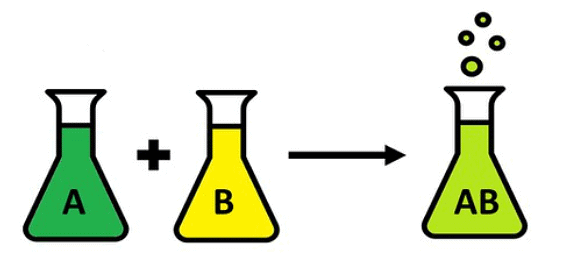A Chemical Reaction

Q5: What is a balanced chemical equation?
Ans: A balanced chemical equation is one in which the number of atoms involved on the reactant side is equal to the number of atoms on the product side. This is based on the law of conservation of mass which states that matter cannot be created or destroyed. For example, the balanced chemical equation for the reaction between hydrogen and oxygen to form water is 2H2 + O2 → 2H2O.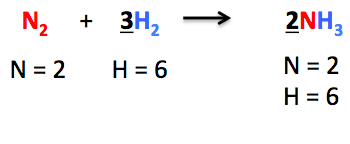A Balanced Chemical Equation

Q6: Why is it necessary to balance a chemical equation?
Ans:

• Balancing a chemical equation is essential because it follows the Law of Conservation of Mass, which states that the total mass of reactants should be equal to the total mass of the products in a chemical reaction.
• This law implies that the number of atoms for each element in the reactants side should be the same as the number of atoms for that element in the products side.
• Without balance, the chemical equation may incorrectly represent the actual reaction, leading to inaccurate predictions about the quantities of reactants needed or products formed.

Q7: What is the method of balancing chemical equations?
Ans: The hit and trial method is used for balancing simple chemical equations. In this method, coefficients before the symbols/formulae of the reactants and products are adjusted in such a way that the total number of atoms of each element on both sides becomes equal.

For example, consider the unbalanced equation

H2 + O2   H2O

Here, there are 2 H atoms on the left and only 1 on the right, and 2 O atoms on the left but only 1 on the right. By adjusting coefficients, we get the balanced equation as

2H2 + O2   2H2O

Now, there are 4 H atoms and 2 O atoms on both sides.

Q8: Can rancidity retard by storing foods away from the light?
Ans: Rancidity is a process whereby food products, particularly those containing fats and oils, deteriorate in quality, leading to unpleasant smells and tastes. This occurs when the fats and oils within the food oxidize over time. If food is stored away from light, this oxidation process slows down, therefore retarding the development of rancidity. Thus, proper storage can significantly delay the onset of this undesirable characteristic.

Q9: In the equations given below, state giving reasons, for whether substances have been oxidized or reduced.
(i) PbO + CO → Pb + CO2
(ii) H2S + Cl2 → 2HCl + S

Ans:
(i) Carbon monoxide is oxidized as it gains oxygen.
(ii) Chlorine is reduced as it gains hydrogen.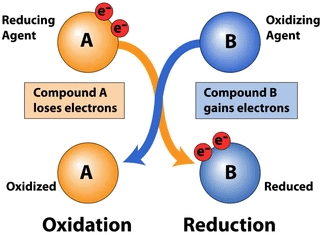Q10: A sample of water weed was placed in water and exposed to sunlight. Bubbles of gas are seen on the surface of the leaves.
(i) Name the gas evolved.
(ii) Name the process taking place.
(iii) Write a balanced equation of reaction taking place.
Ans:

(i) Oxygen
(ii) Photosynthesis
(iii) Carbon Dioxide + Water→Glucose + Oxygen

that means,

6CO2 + 6H2O → C6H12O6 + 6O2

Q11: Give balanced equations, wherever possible, or where this is not possible, explain the following by means of examples:
(i) A reaction which gives out heat.
(ii) A reaction that takes place with the help of sunlight.
(iii) A reaction that is brought about by the electric current.
(iv) A reversible reaction.
(v) A reaction with a solid and gas that produces heat.
Ans:

(i) Coke on heating in air, catches fire and liberates a large amount of heat.
C + O2 → CO2 + Heat
(ii) Carbon dioxide and water react in the presence of chlorophyll and sunlight to form glucose and oxygen.
6CO2 + 6H2O  → C6H12O6 + 6O2
(iii) Molten lead bromide decomposes into lead metal and bromide on the passage of electric current.
PbBr2 → Pb + Br2
(iv) The reaction between red hot iron and steam is reversible.
3Fe + 4H2O ⇌ Fe3O4 + 4H2
(v) When magnesium burns in air or oxygen it liberates a large amount of heat.
2Mg + O2  → 2MgO + Heat.

Q12: Balance the following chemical equations:
(i) Mg + N2 → Mg3N2
(ii) KClO3 → KCL + O2
Ans:

(i) Mg + N2 → Mg3N2
Looking at the unbalanced equation, we see that we have one magnesium (Mg) atom on the reactant side and three on the product side (in Mg3N2). To balance this, we add a coefficient of 3 in front of Mg on the reactant side:
3Mg + N2 → Mg3N2
Now, we check the nitrogen (N) atoms. We have two nitrogen (N) atoms on both sides (in N2 and Mg3N2), so the nitrogen is already balanced.

(ii) KClO3 → KCl + 3O2.

To balance the equation, we place 2 in front of KClO3 on the left side and 2 in front of KCl on the right side. Now, the K and Cl atoms are balanced on both sides.
Next, we place 3 in front of O2 on the right side to balance the O atoms. Now, the number of O atoms is the same on both sides. Thus, the balanced equation is

2KClO3 → 2KCl + 3O2.

Q13: Write the uses of decomposition reactions.
Ans:

• Metal Extraction: They play a vital role in extracting metals like aluminum, sodium, and potassium from their compounds in ores through processes like electrolysis.
• Chemical Synthesis: Decomposition reactions are employed to create specific chemicals and compounds by breaking down complex substances.
• Environmental Applications: In waste treatment and pollution control, decomposition reactions help break down harmful substances into less toxic forms.
• Food Preparation: Baking soda (sodium bicarbonate) decomposes to release carbon dioxide, making dough rise in baking.
• Explosives: Decomposition reactions are involved in explosive devices, releasing energy rapidly.

Q14: What are the characteristics of chemical reactions?
Ans:

Characteristics of chemical reactions are as follows:
(i) Evolution of a gas
(ii) Formation of a precipitate
(iii) Change in color
(iv) Change in temperature
(v) Change in the state.

Q15: What are the different ways that can make chemical equations more informative
(i) By indicating the “physical states” of reactants and products.
(ii) By indicating the “heat changes” taking place in the reaction.
(iii) By indicating the “conditions” under which the reaction takes place.

The document Short Answer Questions: Chemical Reactions & Equations | Science Class 10 is a part of the Class 10 Course Science Class 10.
All you need of Class 10 at this link: Class 10

## Science Class 10

78 videos|509 docs|153 tests

## FAQs on Short Answer Questions: Chemical Reactions & Equations - Science Class 10

 1. What is a chemical reaction?Ans. A chemical reaction is a process in which one or more substances, known as reactants, are transformed into different substances, known as products, by breaking and forming chemical bonds.
 2. What are the different types of chemical reactions?Ans. There are several types of chemical reactions, including combination reactions, decomposition reactions, displacement reactions, redox reactions, and acid-base reactions. Each type has its own characteristics and involves different changes in chemical bonds.
 3. How are chemical reactions represented?Ans. Chemical reactions are represented using chemical equations. In a chemical equation, the reactants are written on the left side, and the products are written on the right side. The reactants and products are separated by an arrow, indicating the direction of the reaction.
 4. What is the importance of balancing chemical equations?Ans. Balancing chemical equations is important because it ensures that the law of conservation of mass is followed. This law states that matter cannot be created or destroyed in a chemical reaction, only rearranged. Balancing equations ensures that the number of atoms of each element is the same on both sides of the equation.
 5. How can one identify a chemical reaction?Ans. There are several signs that indicate a chemical reaction has occurred. Some common signs include the formation of a precipitate (a solid that forms from a liquid mixture), the evolution of gas (bubbles or a change in odor), a change in color, the release or absorption of heat, or the production of light.

## Science Class 10

78 videos|509 docs|153 testsExplore Courses for Class 10 examSignup to see your scores go up within 7 days! Learn & Practice with 1000+ FREE Notes, Videos & Tests.
10M+ students study on EduRev
Track your progress, build streaks, highlight & save important lessons and more!
Related Searches

,

,

,

,

,

,

,

,

,

,

,

,

,

,

,

,

,

,

,

,

,

;